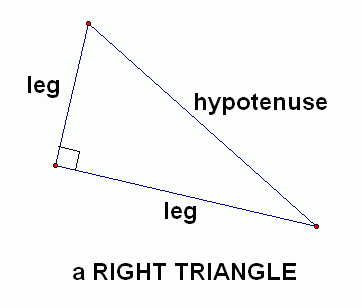Search
• Lola agbetunsin

# Geometry: Pythagorean Triples

Geometry: Pythagorean triples (memorize for success)

This is for 7th graders, 8th graders and geometry students, and SAT test takersPythagorean triples are integer side lengths of a right triangle. The biggest number is the hypotenuse, and the two smaller numbers are the legs of the triangle.

3,4, 5 5,12,13 8,15,17 7, 24, 25 and there multiples like 6, 8,10 10, 24, 26

Example 1: If the two legs of a right angle triangle are 3cm and 4cm, what is the value of the hypotenuse?

Example 2 if the diagonal of a rectangle is 25 in, and the length of the rectangle is 24 in, what is the width of the rectangle?

Hint: a diagonal cuts a rectangle into two equal right triangles whose hypotenuse is the diagonal. sketch the rectangle